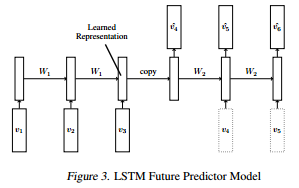# Doubt regarding LSTM unsupervised future predictor input

According to the tutorial in https://pytorch.org/tutorials/intermediate/seq2seq_translation_tutorial.html in the training part, the input is passed one word at a time, can I pass the entire sentence instead arranged as (seq_len,batch_size,feature_dim) ?

I am trying to implement the model given in this paperI implemented it by using two lstm blocks connected to a buffer linear layer.

I have a input sequence to the encoder of size (3,1,8) where 3 is the sequence length,

I pass it as ec(input_sequence) to get the cell state and the hidden state

according to the paper i need to copy the hidden state to the future predictor, which is fine. What i dont understand is the input to the future predictor. The paper says that the lstm can be conditioned or unconditioned on the last generated frame.

If I chose the unconditioned path, do I just pass a sequence of zero vectors ?

If I chose the conditioned path, do I pass a sequence of vectors intended to be the future vectors as input ? If this is the case, wouldnt the inference situation be a case where I pass the current sequence and future sequence to get the future sequence ? which seems like cheating to me…